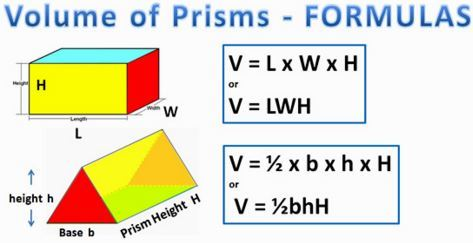Volume of Prisms
3 years ago
mccraryk
Save
Edit
Host a game
Live GameLive
Homework
Solo Practice
Practice15 QuestionsShow answers
• Question 1
30 seconds
Q. What is volume?
The amount of space of a 2-D object
The amount of space in a 3-D object
Water
Area
• Question 2
30 seconds
Q. B represents...
Area of Base
Base
Bottom
Area of Bridge
• Question 3
30 secondsQ. This formula finds the volume of what figures?
Rectangular Prisms
Triangular Prisms
Cylinders
All 3-D figures
• Question 4
120 secondsQ. This square prism has a volume of 1600mm3. What is it's width?
8mm
64mm
32mm
25mm
• Question 5
180 secondsQ. Find the volume of the figure.
63 cm³
91 cm³
819 cm³
409.5 cm³
• Question 6
180 secondsQ. Find the volume of the figure. Round to the nearest tenth.
80 cm³
40 cm²
80 cm2
40 cm³
• Question 7
120 secondsQ. This cube has a volume of 216 in3. What is the length of a side?
8.67 in
6 in
108 in
36 in
• Question 8
180 secondsQ. Find the volume of the figure. Round to the nearest whole number.
1,944 cm³
108 cm³
972 cm³
216 cm³
• Question 9
180 secondsQ. Find the volume of the figure.
150 in³
100 in³
300 in³
180 in³
• Question 10
120 secondsQ. If this prism has a volume of 220ft3, what would it's height need to be?
2ft
3ft
10ft
11ft
• Question 11
900 seconds
Q. 10. A fish-tank has a length of 45 centimeters, a width of 25 centimeters and a depth of 10 centimeters. Find the volume of the fish-tank.
10250 cm3
11250 cm3
10000cm3
11050 cm3
• Question 12
60 secondsQ. What is the volume?
72 units3
27 units3
1.5 units3
272 units3
• Question 13
120 seconds
Q. The volume of a rectangular prism is 125 units 3.  If the height is 25 units, what is the area of the base?
5 units2
3,125 units2
15 units2
25 units2
• Question 14
120 secondsQ. The volume of this prism is 540m3.  Find the base of the triangle.
2.5m
12m
5m
10m
• Question 15
120 secondsQ. This isosceles right triangular prism has a volume of 2058cm3.  Find the height of the triangle.